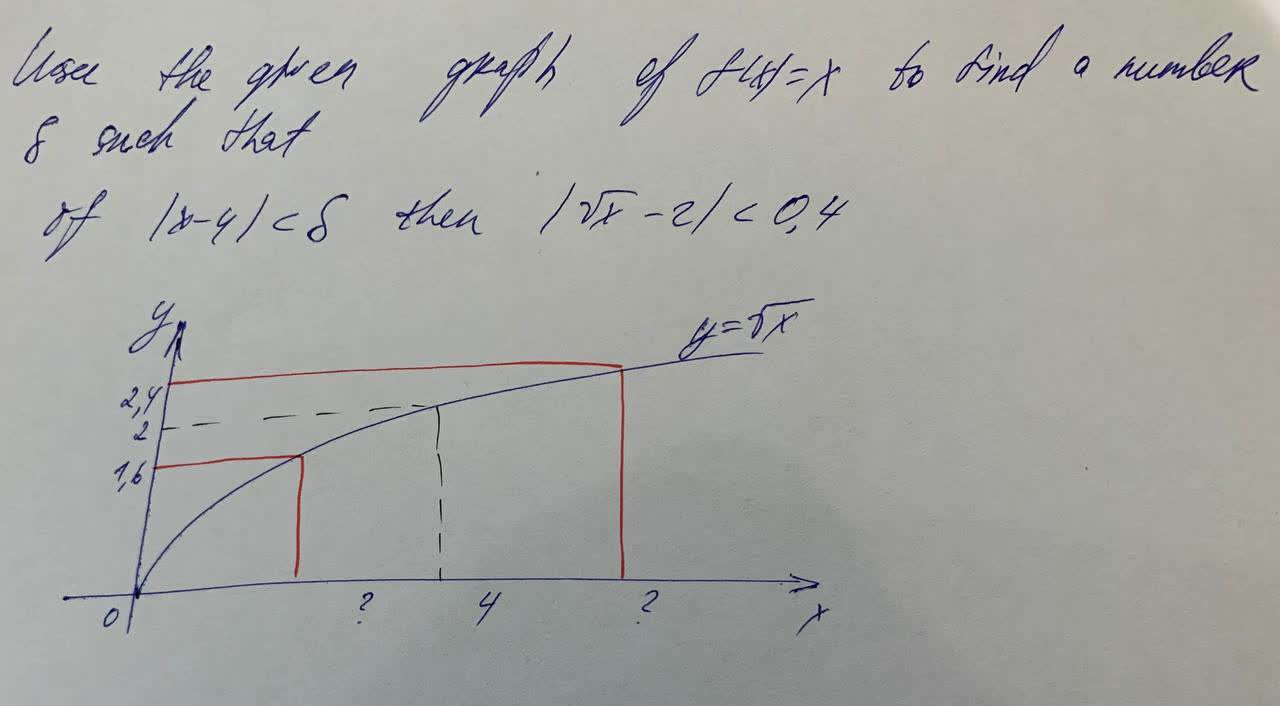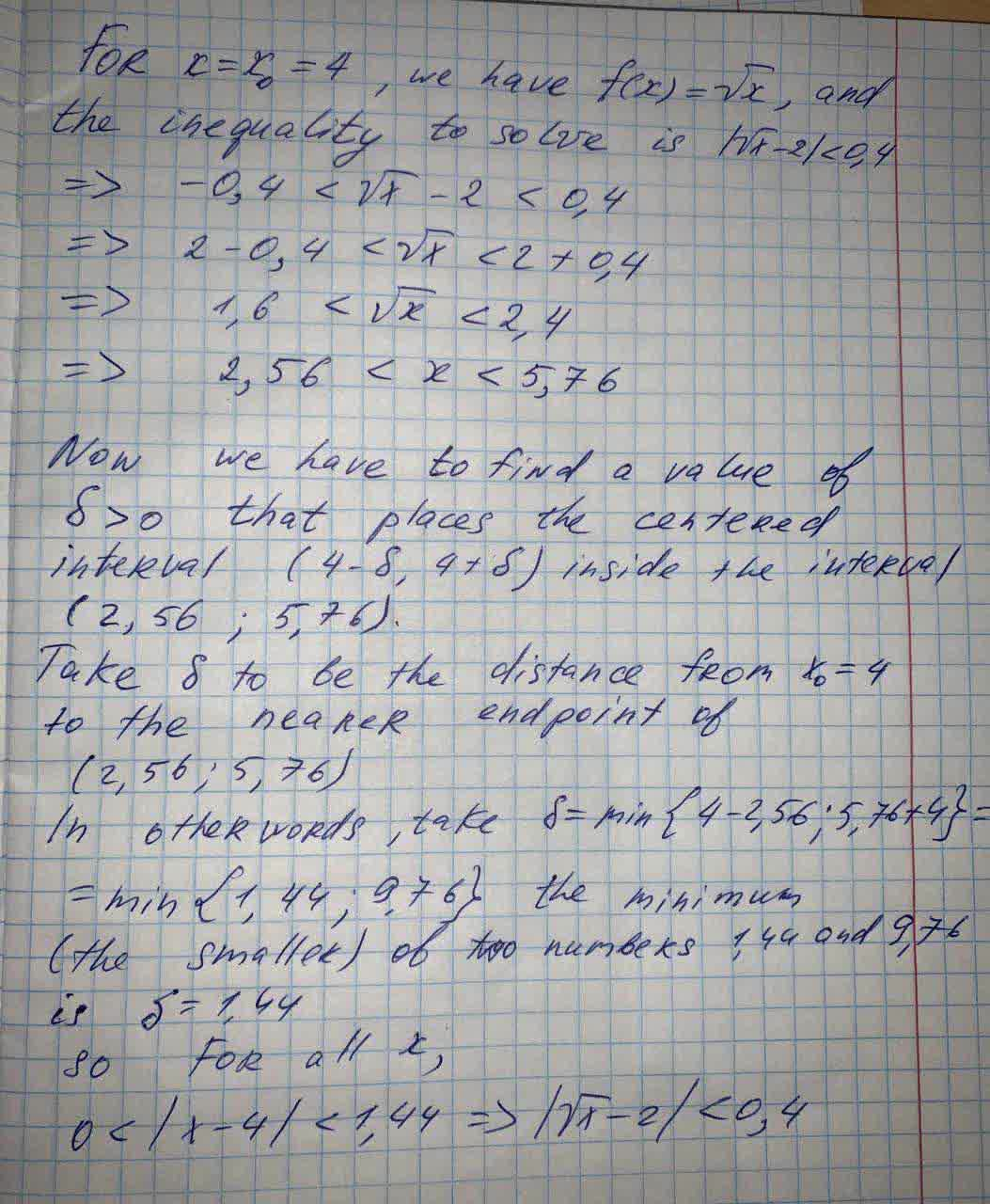Question# Use this graph to find a number of g such that

Linear equations and graphs
ANSWEREDUse this graph to find a number of g such that